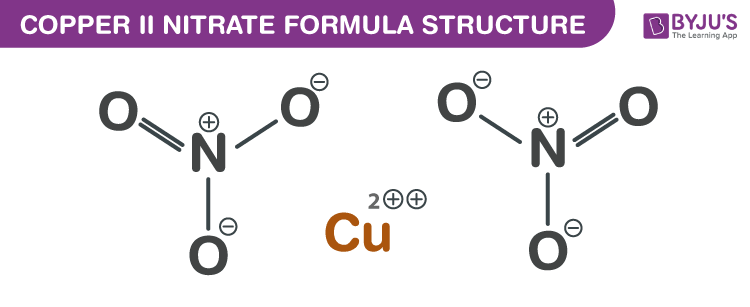# Copper II Nitrate Formula

Copper II Nitrate formula, also known as Cupric nitrate formula or Copper Dinitrate formula is explained in this article. This inorganic compound occurs in the form of a blue crystalline solid. The molecular or chemical formula of Copper II Nitrate is Cu(NO3)2.

Cupric nitrate can be obtained in two forms viz as a hexahydrate and as a trihydrate. Copper Dinitrate is widely used in the production of medicines, in chemical analysis, as an insecticide, and in the making of light-sensitive papers. On burning this compound it produces toxic oxides of nitrogen.

## Copper II Nitrate Formula Structure## Properties Of Copper II Nitrate

 Chemical formula Cu(NO3)2 Molecular weight 187.5558 g/mol (anhydrous) Density 3.05 g/cm3 (anhydrous) Boiling point Sublimes Melting point 256 °C (anhydrous)